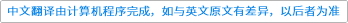Communications in Mathematical Physics ( IF 2.102 ) Pub Date : 2021-04-07 , DOI: 10.1007/s00220-021-04069-z
Michael Röckner, Longjie Xie

We study the asymptotic behavior for an inhomogeneous multiscale stochastic dynamical system with non-smooth coefficients. Depending on the averaging regime and the homogenization regime, two strong convergences in the averaging principle of functional law of large numbers type are established. Then we consider the small fluctuations of the system around its average. Nine cases of functional central limit type theorems are obtained. In particular, even though the averaged equation for the original system is the same, the corresponding homogenization limit for the normal deviation can be quite different due to the difference in the interactions between the fast scales and the deviation scales. We provide quite intuitive explanations for each case. Furthermore, sharp rates both for the strong convergences and the functional central limit theorems are obtained, and these convergences are shown to rely only on the regularity of the coefficients of the system with respect to the slow variable, and do not depend on their regularity with respect to the fast variable, which coincide with the intuition since in the limit equations the fast component has been totally averaged or homogenized out.down
wechat
bug